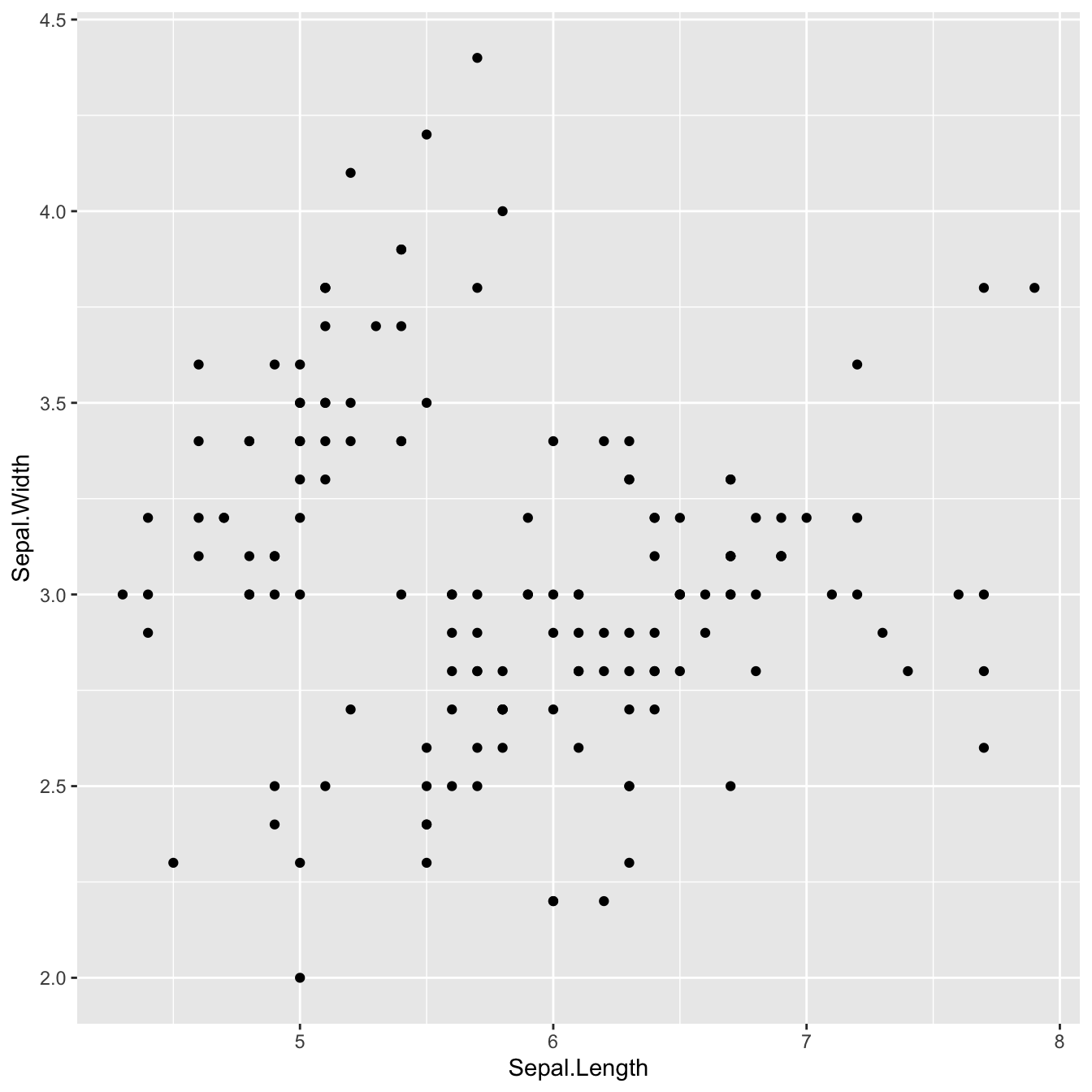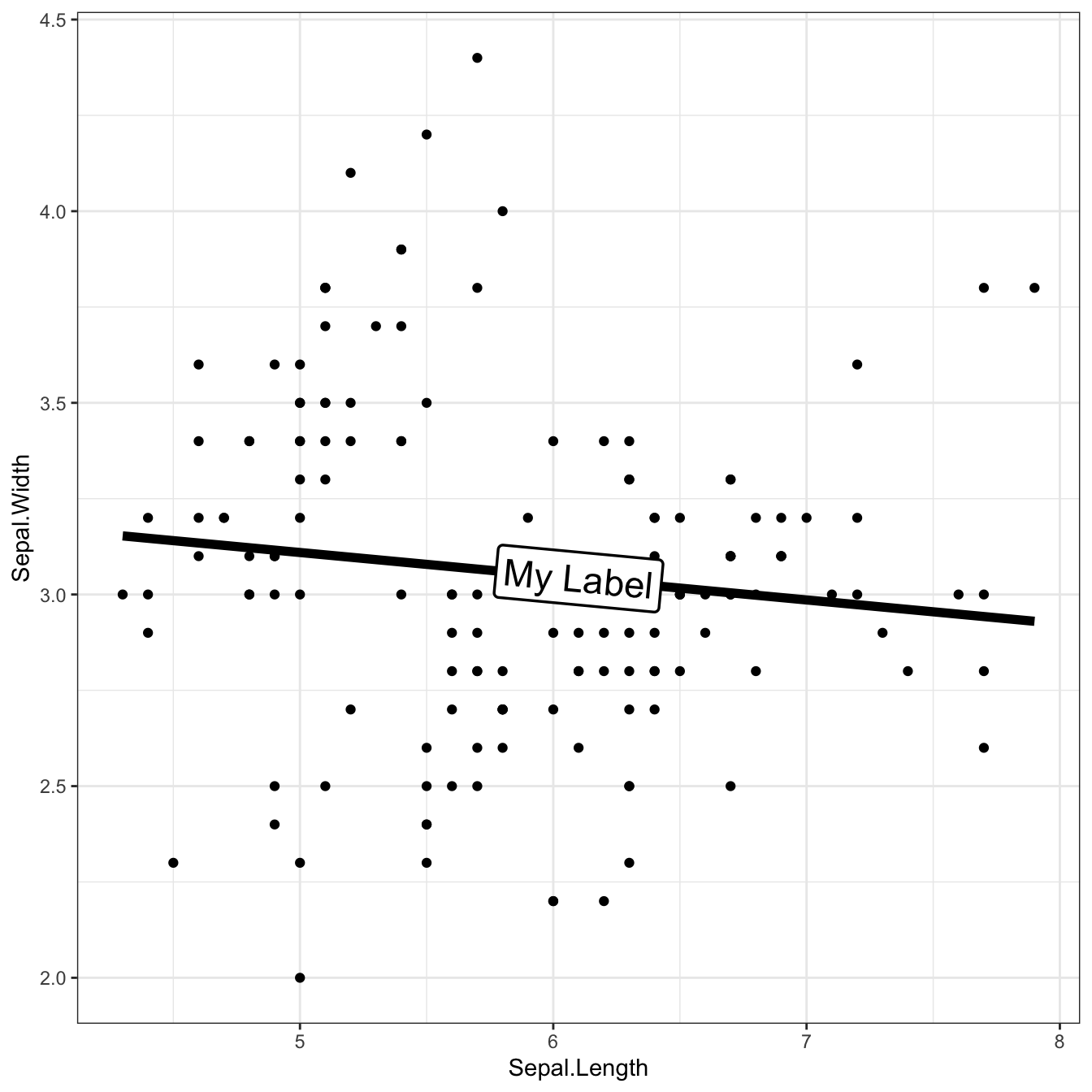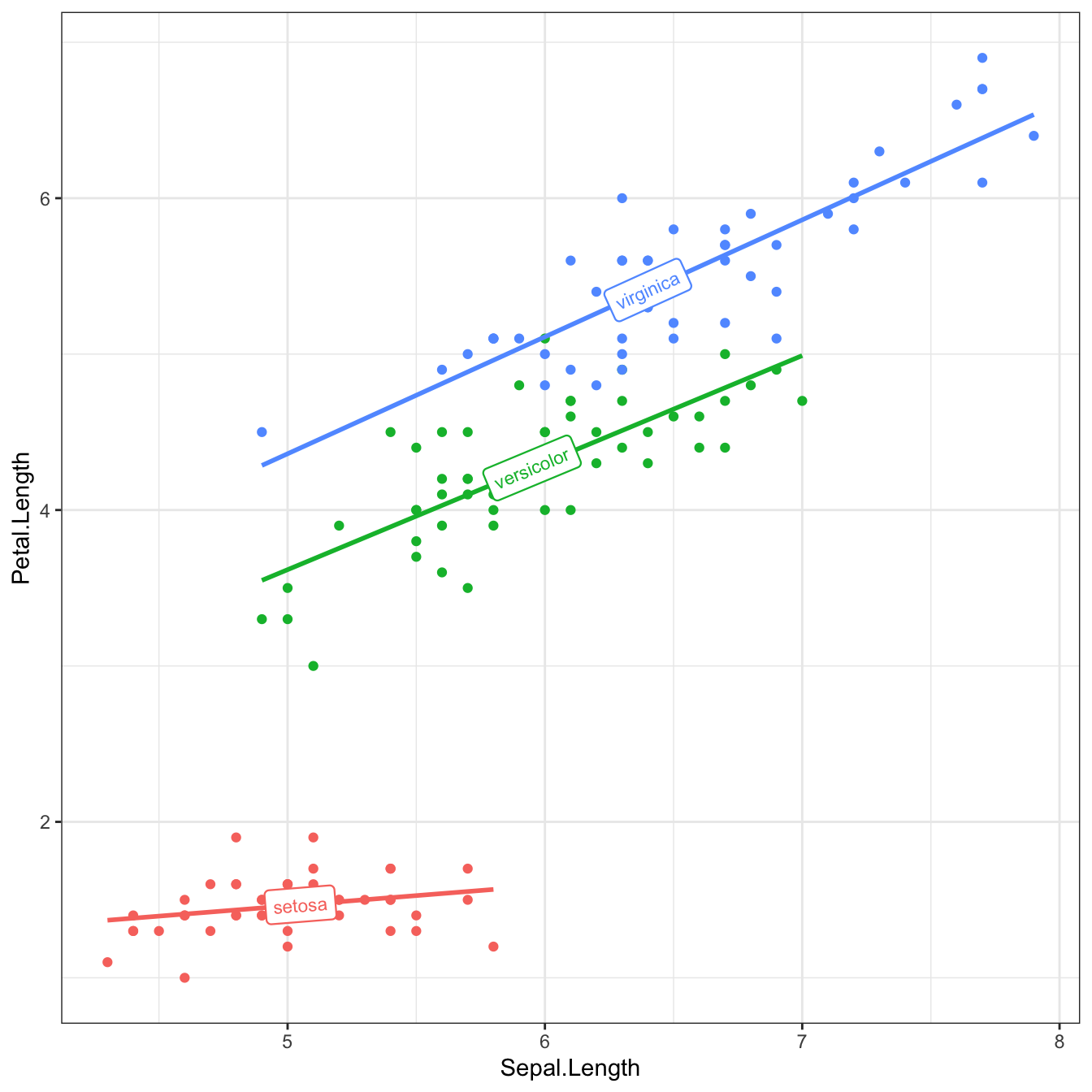# Scatter plot with trend lines and labels on top

This post explains how to add a text or labels on the trend line in a scatter with one or multiple groups. It uses the ggplot2 and geomtextpath packages for creating the chart and making it nice.

# Package

For this post, we need the ggplot2 and geomtextpath packages.

``````# Install the packages if not already done
# install.packages("ggplot2")
# install.packages("geomtextpath")

library(ggplot2)
library(geomtextpath)``````

# Default scatter plot in ggplot2

Here’s what the default scatter plot output looks like with `ggplot2`:# Add trend line with label on top

With the `geom_labelsmooth()` function, we add a trend line with a label on it!

It has the following arguments:

• `fill`: the background color of the label

• `method`: type of trend wanted. In our case, `lm` means the ordinary least squared estimator (linear regression). Check the function documentation for available possibilities

• `size`, `linewidth` and `boxlinewidth`: define the properties of the text and its box

``````library(hrbrthemes)
data(iris)

ggplot(iris, aes(x = Sepal.Length, y = Sepal.Width)) +
geom_point() +
geom_labelsmooth(aes(label = 'My Label'), fill = "white",
method = "lm", formula = y ~ x,
size = 6, linewidth = 2, boxlinewidth = 0.6) +
theme_bw() + guides(color = 'none') # remove legend``````# Multiple groups trend lines with labels

In the case of a multi-group scatter plot, the `geom_labelsmooth()` function works just as simply:

• we add `color=Species`

• we change `label = 'My Label'` to `label = Species`

And that’s it!

``````library(hrbrthemes)
data(iris)

ggplot(iris, aes(x = Sepal.Length, y = Petal.Length, color = Species)) +
geom_point() +
geom_labelsmooth(aes(label = Species), fill = "white",
method = "lm", formula = y ~ x,
size = 3, linewidth = 1, boxlinewidth = 0.4) +
theme_bw() + guides(color = 'none') # remove legend``````# Conclusion

In this post, we look at how to use the geomtexpath package to create scatter plots with trend lines and labels. To find out more about how to customize a scatter plot, see the dedicated section.

Related chart types

## Contact

This document is a work by Yan Holtz. Any feedback is highly encouraged. You can fill an issue on Github, drop me a message on Twitter, or send an email pasting yan.holtz.data with gmail.com.